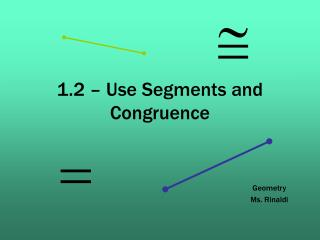Download Presentation1.2 – Use Segments and Congruence1.2 – Use Segments and Congruence - PowerPoint PPT Presentation

Download Presentation1.2 – Use Segments and Congruence
An Image/Link below is provided (as is) to download presentation

Download Policy: Content on the Website is provided to you AS IS for your information and personal use and may not be sold / licensed / shared on other websites without getting consent from its author. While downloading, if for some reason you are not able to download a presentation, the publisher may have deleted the file from their server.

- - - - - - - - - - - - - - - - - - - - - - - - - - - E N D - - - - - - - - - - - - - - - - - - - - - - - - - - -
Presentation Transcript

1. 1.2 – Use Segments and Congruence Geometry Ms. Rinaldi

2. Postulate In Geometry, a rule that is accepted without proof is called a postulate or axiom. Postulate 1 – The Ruler Postulate

3. Measure the length of STto the nearest tenth of a centimeter. The length of STis about 3.4 centimeters. ANSWER EXAMPLE 1 Apply the Ruler Postulate

4. 1 8 a. ANSWER 5 1 in. 8 b. ANSWER 1 in. 3 8 Apply the Ruler Postulate EXAMPLE 2 Use a ruler to measure the length of the segment to the nearest inch.

5. Definition • Between –

6. Postulate 2 – Segment Addition Postulate

7. Maps The cities shown on the map lie approximately in a straight line. Use the given distances to find the distance from Lubbock, Texas, to St. Louis, Missouri. The distance from Lubbock to St. Louis is about 740 miles. ANSWER EXAMPLE 3 Apply the the Segment Addition Postulate SOLUTION Because Tulsa, Oklahoma, lies between Lubbock and St. Louis, you can apply the Segment Addition Postulate. LS = LT + TS = 380 + 360 = 740

8. In Exercises 3 and 4, use the diagram shown. In the diagram, WY = 30. Can you use the Segment Addition Postulate to find the distance between points Wand Z? Explain your reasoning. b. a. Use the Segment Addition Postulate to find XZ. ANSWER 73 ANSWER No; W is not between X and Z. Find a length EXAMPLE 4

9. Use the diagram to find GH. FH = FG+ GH 36 21+GH = 15 GH = EXAMPLE 5 Find a length SOLUTION Use the Segment Addition Postulate to write an equation. Then solve the equation to find GH. Segment Addition Postulate. Substitute 36 for FHand 21 for FG. Subtract 21 from each side.

10. Use the diagram to find WX. ANSWER 107 Find a length EXAMPLE 6

11. Definition • Congruent Segments –

12. Plot J(– 3, 4), K(2, 4), L(1, 3), and M(1, – 2) in a coordinate plane. Then determine whether JKand LMare congruent. EXAMPLE 7 Compare segments for congruence

13. Plot the points A(– 2, 4),B(3, 4), C(0, 2), and D(0, – 2) in a coordinate plane. Then determine whether ABand CDare congruent. Compare segments for congruence EXAMPLE 8

14. Homework Pages 12 – 13 # 1, 6-14, 20, 21-23